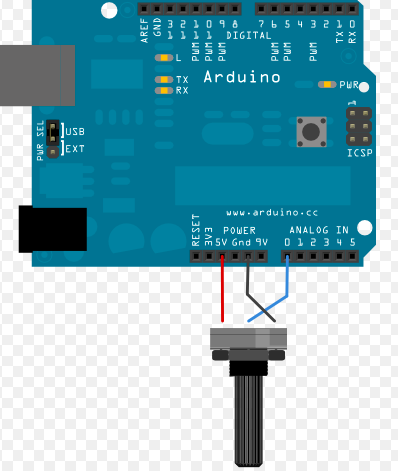﻿ How to use a potentiometer connected to arduino to increment and decrement a variable. It includes also the description of the map function used to remap range of integers value

# Potentiometer

How to use a potentiometer connected to arduino to increment and decrement a variable. It includes also the description of the map function used to remap range of integers value

A potentiometer is simply a knob which is able to modify the value of a resistance. A resistance value is an analog value that can be read on any analogue pin of Arduino. In the multidimensional box I used a variable resistance (a potentiometer) to change the value of the variable used to define the max distance to use to fine tune the sonic sensor. To use a potentiometer we need to connect three wires to the Arduino board. The first goes to ground from one of the outer pins of the potentiometer. The second goes from 5 volts to the other outer pin of the potentiometer. The third goes from analog input 2 to the middle pin of the potentiometer. By turning the shaft of the potentiometer, we change the amount of resistence on either side of the wiper which is connected to the center pin of the potentiometer. This changes the relative "closeness" of that pin to 5 volts and ground, giving us a different analog input. When the shaft is turned all the way in one direction, there are 0 volts going to the pin, and we read 0. When the shaft is turned all the way in the other direction, there are 5 volts going to the pin and we read 1023. In between, analogRead() returns a number between 0 and 1023 that is proportional to the amount of voltage being applied to the pin.

NOTE: Be careful to not confuse potentiometer with Digital knobs.

Following a possible schema to connect a potentiometer:In order to read a potentiometer start declaring an integer value that we will call: PotValue

``````
int PotValue;
``````

Simply reading the analog value from the specific pin connected to the potentiometer, we will be able to retrive the resistance.

``````
``````
in this case, POTENTIOMETER_PIN is the PIN 0 and is the pin we used to connect the center connector of the potentiometer.
The value returned should be a avalue between 0 and 1023 based on the voltag applied (and cut) on the analog pin. This is valid both for 5V pins or for 3.3 pins like the ones available on the arduino 101 board.
If you want to map your value within a different range (for example 0,100), you do not need to write a complex function. You can simply use an existing function called map which can be very useful in most cases.

``````
NewValue = map(PotValue, 0, 1023, 0, 100);
``````

The prototype of this function is the following:

map(value, fromLow, fromHigh, toLow, toHigh)

This function 'Re-maps' a number from one range to another. That is, a value of fromLow would get mapped to toLow, a value of fromHigh to toHigh, values in-between to values in-between, etc. Does not constrain values to within the range, because out-of-range values are sometimes intended and useful.
The "lower bounds" of either range may be larger or smaller than the "upper bounds" so the map() function may be used to reverse a range of numbers.

``````
y = map(x, 1, 50, 50, 1);
``````

Will invert the number within the same range.

The function also handles negative numbers. For example:

``````

y = map(x, 1, 50, 50, -100);
``````

Will invert and remap in a potential negative value

An interesting usage for potentiometers is to store phisically values which must be used as configuration parameters. For example, I used different potentiometers also to fine tune the algorithm I wrote for the Arduporter Robot. In Arduporter it is really important to fine tune certain parameters like the min distance from walls and the speed to use in certain conditions. Using potentiometers you can increment or decrement variables in the code and use those values as real configuration parameters.

The following code represents a possible function that you can add to your code in order to use a potentiometer as a phisical variable to store a "minDistance" value to use for your robotics applications

``````
void CheckPotentiometer()
{

char buf;

unsigned long currentMillis = millis();

if (currentMillis - previousMillis > potentiometerInterval) {

previousMillis = currentMillis;

MinDistance = map(analogRead(A4), 0, 690, 0, 20);

if (oldMinDistance != MinDistance) {
sprintf(buf, "MinDistance changed to: %d", MinDistance);
tft.drawString(buf, 0, yDraw, 2);
yDraw = scroll_line();
oldMinDistance = MinDistance;
}
}
}
``````